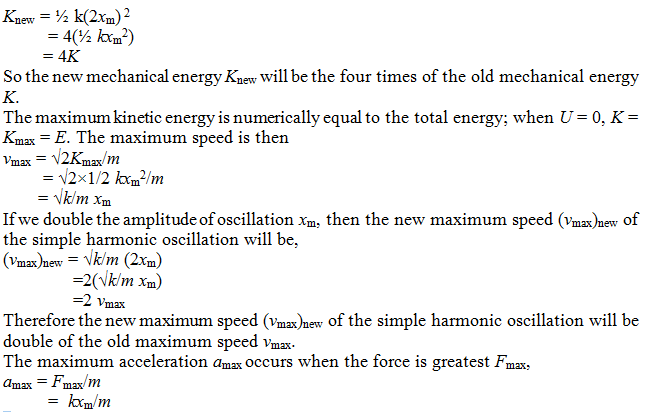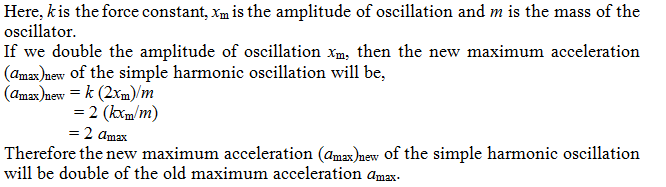Click to Chat

1800-1023-196

+91-120-4616500

CART 0

• 0

MY CART (5)

Use Coupon: CART20 and get 20% off on all online Study Material

ITEM
DETAILS
MRP
DISCOUNT
FINAL PRICE
Total Price: Rs.

There are no items in this cart.
Continue Shopping

How are each of the following properties of a simple harmonic oscillators affected by doubling the amplitude; period, force constant, total mechanical energy, maximum velocity, maximum acceleration?
4 years ago

Time period (T) of simple harmonic oscillation is defined as,
T = 2π √m/k
Here, m is the mass of the oscillator and k is the force constant.
From the above equation, T = 2π √m/k we observed that, the time period (T) of simple harmonic oscillation does not depend upon the amplitude of oscillation xm. Therefore the time period (T) of simple harmonic oscillation will not affected if we the doubling the amplitude of the oscillation.
Total mechanical energy (K) of the simple harmonic oscillation is defined as,
K = ½ kxm2
Here, k is the force constant and xm is the amplitude of oscillation.
If we double the amplitude of oscillation xm, then the new mechanical energy (Knew) of the simple harmonic oscillation will be,4 years ago
Think You Can Provide A Better Answer ?
Answer & Earn Cool Goodies

Other Related Questions on Wave Motion

View all Questions »Course Features

• 101 Video Lectures
• Revision Notes
• Previous Year Papers
• Mind Map
• Study Planner
• NCERT Solutions
• Discussion Forum
• Test paper with Video SolutionCourse Features

• 110 Video Lectures
• Revision Notes
• Test paper with Video Solution
• Mind Map
• Study Planner
• NCERT Solutions
• Discussion Forum
• Previous Year Exam Questions